Spirograph
 What is a Spirograph? Drawing Exercises Formulas Hypocycloids Epicycloids Cycloids Repeating Figures Spirograph on the Internet  References ..
 To the Main Page    "Mathematische Basteleien"

What is a Spirograph?
 ...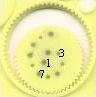... The Spirograph is a mathematical toy, which you can use for drawing nice figures. In the simplest case it exists of a fixed circle, used as a template, and a smaller rolling circle with holes.
Drawing a figure:
Hold the template (clipping). Stick a ballpoint pen through one of the holes of the smaller circle. Roll it inside the bigger fixed circle. Draw it on a sheet of paper. Cogs at the edges of the circles guarantee a reliable unrolling and prevent sliding.
It is a pity that the spirograph introduced here is non-transparent. It was a gift of my savings bank at the "World Saving Day", if I remember correctly.

Drawing Exercises top
 ...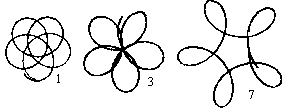... You can draw rosettes with five points easily.  I chose only three of twelve possible holes. They are representative for the possible shapes.
If you look at the figures  more accurately, you see that they aren't closed after five revolutions. This is intended:
 ...... If you go on drawing you'll get some nicely shaped rosettes. This shows the attraction of spirograph figures.

Formulas   top
You can find parameter formulas for the figures, which describe them mathematically.

Names:
 ...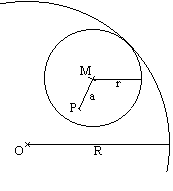... The large circle (radius R) is given and fixed. The small circle (radius r) rolls on its inner side. There is point P fixed on the inner circle.  It has the distance a from the centre M of the small circle. Now you follow the way of  point P during rolling.
Calculations: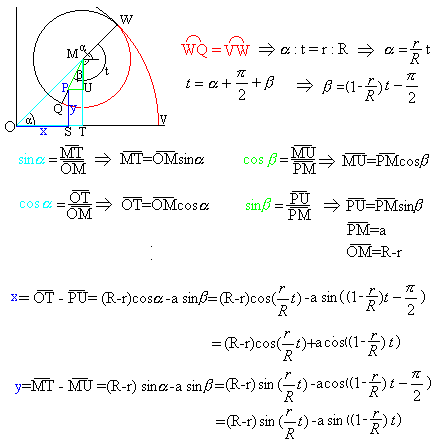...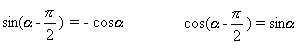... In the end you have to use both trigonometric formulas.
Result:
 ...... You get the parametric equations for the movement of the point on the left.  The coordinates x and y of point P depend on the angle t. The variables R, r and a are constant.
The trigonometric formulas guarantee periodical movements. The variables (R - r) and a determine the height, the ratio (r : R) the periodicity of the drawings.

Hypocycloids  top
If you use a program to draw the graph of the equations, you get the so-called hypocycloids.
 ...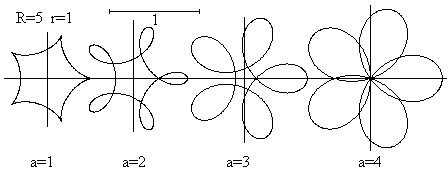... The parameter a is different for each picture.  You also can choose cases, which cannot be drawn with the spirograph, because the point P lies outside the rolling circle.
There are closed curves in difference to the spirograph drawings, because the ratio (R : r) is a digit. It is sufficient to choose the numbers 0 to 5*2Pi for t.

The ratio (R : r) is not a digit, so that you get the graphs from above.
 ...... The shape of a rosette also depends on the number of revolutions.

Epicycloids   top
...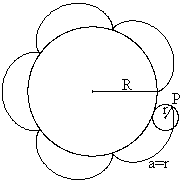... You get another kind of cycloids, if you let unroll the smaller circle (r=1) outside the fixed circle(R=5). This is also realized at the spirograph with two circles.
Parameter equations: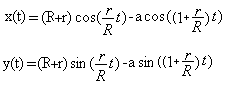If you transfer the equations to graphs with the help of a program, you get so-called epicycloids.... The parameter a is changed.  You also can choose cases, where the point P lies outside the rolling circle.

Cycloids    topIf you roll the circle on a straight line, a fixed point on the circle line describes the cycloid.

The standard equations of the cycloid are x = r[t sin(t) ] and y = r[1 cos(t) ], where r is the radius of the rolling circle and t goes through the numbers from 0 to 2Pi for one period.
It is remarkable that the length of a cycloid is eight times as long as the radius of the producing circle. The surface between x axis and cycloid is three times as big as the surface of the producing circle.

You get more cycloids if the point P writing the cycloid is within or outside the rolling circle.
In the first case a shortened cycloid develops, in the second case an extended one.
The general parametric representation is x = rt-a sin(t) und y =r-acos(t).  R is the radius of the rolling circle and a the distance of the point P of its center.
 ...... There are r=3 and a=1 (blue), a=3 (green) and a=5 (red). the variable t follows 0
A rectangle and two half circles sitting on two opposite sides belong to this part of the spirograph. The drawing is a combined  epicycles / cycloids.A program found parts of the graph on the left with the help of the following parametric equations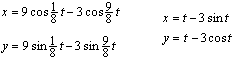Epicycloids equations on the left, cycloids equations on the right.

Repeating Figures top
There is a stencil with simple figures inside and a fixed circle, which also belongs to the spirograph system.
 ...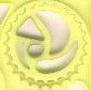... ...... You draw a figure, move the wheel further by one cog and draw the next figure.  You repeat this procedure as long as cogs are there. In the end there is a ring for instance.
These drawings aren't interesting from a mathematical view, but very effective.

You can read more on my German page Epizykloide.

Spirograph on the Internet top

English

Nathan Friend
inspirograph  (Applet)

Richard Parris (Freeware-Programs)
winplot

Wikipedia
Spirograph, Harmonograph

German

Jutta Gut
Zykloiden

Wikipedia
Spirograph (Spielzeug)Zykloide, Epizykloiden

References   top
W.Leupold...: Analysis für Ingenieur- und Fachschulen, Verlag Harri Deutsch, Frankfurt/M.

Feedback: Email address on my main page